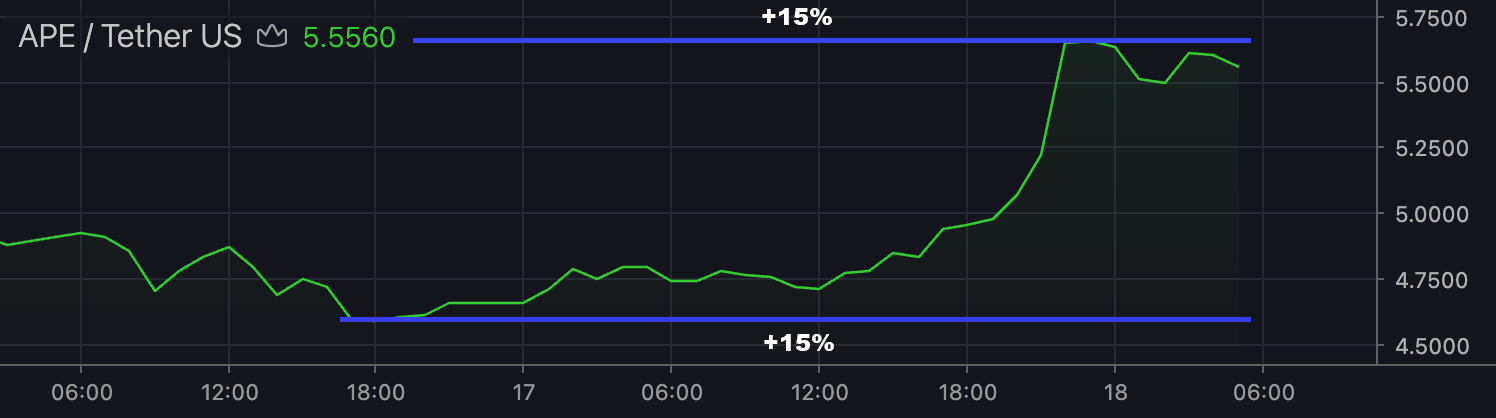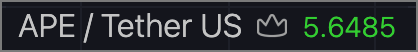# Overlay Scripts ​

Overlay scripts define how your data will look on the chart.

As was described in the Scripts chapter, each `.navy` file contains a javascript code (almost), separated by section tags.

## Basic structure ​

Typical overlay looks like this:

js
``````// Navy ~ 0.1-lite // (1)

[OVERLAY name=NewOverlay, ctx=Canvas, verion=0.1.0] // (2)

// Overlay props
prop('color', { type: 'color', def: '#2d7b2f33' }) // (3)

// Draw call
draw(ctx) { // (4)
const layout = \$core.layout
ctx.fillStyle = \$props.color
ctx.fillRect(0, 0, layout.width, layout.height) // (5)
}

// Legend, defined as pairs [value, color]
legend(x) => [[Math.random(), \$props.color]] // (6)``````
``````// Navy ~ 0.1-lite // (1)

[OVERLAY name=NewOverlay, ctx=Canvas, verion=0.1.0] // (2)

// Overlay props
prop('color', { type: 'color', def: '#2d7b2f33' }) // (3)

// Draw call
draw(ctx) { // (4)
const layout = \$core.layout
ctx.fillStyle = \$props.color
ctx.fillRect(0, 0, layout.width, layout.height) // (5)
}

// Legend, defined as pairs [value, color]
legend(x) => [[Math.random(), \$props.color]] // (6)``````

The typical parts are:

1. NavyJS version comment
2. `OVERLAY` tag defining the meta-information
3. `prop` function call that defines new property
4. `draw` draw function, called every time data/view is changed
5. CanvasJS API call
6. A function defining the overlay behavior

## Functional Interface ​

An overlay behavior can be defined by overriding the following core functions.

### Life-cycle ​

js
``````init() // Called when overlay is created
destroy() // Called when overlay is destroyed``````
``````init() // Called when overlay is created
destroy() // Called when overlay is destroyed``````

### Drawing functions ​

js
``````draw() // Main drawing call
drawSidebar() // Drawing call of sidebar
drawBotbar() // Drawing call of botbar``````
``````draw() // Main drawing call
drawSidebar() // Drawing call of sidebar
drawBotbar() // Drawing call of botbar``````

### Samplers / transforms ​

js
``````yRange() // Redefines y-range of overlay
preSampler() // Defines a sampler for precision detection algo
ohlc() // Mapping data point to OHLC value for magnets``````
``````yRange() // Redefines y-range of overlay
preSampler() // Defines a sampler for precision detection algo
ohlc() // Mapping data point to OHLC value for magnets``````

### UI functions ​

js
``````legend() // Defines legend as [value, color] pair
legendHtml() // Defines legend as a custom html
valueTracker() // Sets price label + price line``````
``````legend() // Defines legend as [value, color] pair
legendHtml() // Defines legend as a custom html
valueTracker() // Sets price label + price line``````

### Mouse listeners ​

js
``````mousemove() // Called on 'mousemove' event
mouseout() // Called on 'mouseout' event
mouseup() // Called on 'mouseup' event
mousedown() // Called on 'mousedown' event
click() // Called on 'click' event``````
``````mousemove() // Called on 'mousemove' event
mouseout() // Called on 'mouseout' event
mouseup() // Called on 'mouseup' event
mousedown() // Called on 'mousedown' event
click() // Called on 'click' event``````

### Keyboard listeners ​

js
``````keyup() // Called on 'keyup' event
keydown() // Called on 'keydown' event
keypress() // Called on 'keypress' event``````
``````keyup() // Called on 'keyup' event
keydown() // Called on 'keydown' event
keypress() // Called on 'keypress' event``````

All the definitions described in NavyJS API.

## Environment ​

Overlay Eviromenmet is a group of variables describing the current chart state. They contain all the information needed to build an overlay.

### \$core ​

Collection of all core elements and other variables, such as `data`, `range` and `view`. You can find all the definitions in NavyJS API as well. The most common use case of `\$core` is the main drawing loop:

js
``````// A fragment of code from Spline.navy:
draw() {
// ...
ctx.beginPath()
const layout = \$core.layout
const data = \$core.data // Full dataset
const view = \$core.view // Visible view
const idx = \$props.dataIndex
for (var i = view.i1, n = view.i2; i <= n; i++) {
let p = data[i]
let x = layout.ti2x(p, i) // Mapping functions
let y = layout.value2y(p[idx]) // ...
ctx.lineTo(x, y)
}
ctx.stroke()
}``````
``````// A fragment of code from Spline.navy:
draw() {
// ...
ctx.beginPath()
const layout = \$core.layout
const data = \$core.data // Full dataset
const view = \$core.view // Visible view
const idx = \$props.dataIndex
for (var i = view.i1, n = view.i2; i <= n; i++) {
let p = data[i]
let x = layout.ti2x(p, i) // Mapping functions
let y = layout.value2y(p[idx]) // ...
ctx.lineTo(x, y)
}
ctx.stroke()
}``````

### \$props ​

Overlay props (`props` field of OverlayObject). Props defined with `prop()` function can be immediately accessed through `\$props` variable:

js
``````prop('color', { type: 'color', def: '#2d7b2f33' })
console.log(\$props.color) // #2d7b2f33``````
``````prop('color', { type: 'color', def: '#2d7b2f33' })
console.log(\$props.color) // #2d7b2f33``````

If a property defined in the `props` field, it will be used by the script (instead of default one).

### \$events ​

Events component. Nothing less, nothing more.

TIP

Hi there! Are you tired of debugging overlays with CodeSadBugs??? Or your own buggy DIY npm apps? Then try our powerful Overlay Studio! Click this link RIGHT NOW and get -100% discount! What are you waiting for? Click the *** **** link! 🔥🔥🔥

### \$lib ​

Collection of primitives & helper functions. For example a single candle can be drawn with a few calls:

js
``````/* CandleDescriptor {
x, // x-coordinate
w, // width
o, h, l, c, // y-coords
green, // true/false
src // Data point ref
}*/
\$lib.candleWick(ctx, candleDescriptor, layout)
\$lib.candleBody(ctx, candleDescriptor, layout)``````
``````/* CandleDescriptor {
x, // x-coordinate
w, // width
o, h, l, c, // y-coords
green, // true/false
src // Data point ref
}*/
\$lib.candleWick(ctx, candleDescriptor, layout)
\$lib.candleBody(ctx, candleDescriptor, layout)``````

## Y-range function ​

Sometimes you need to define a y-range that is different from the one calculated by the library.

INFO

The default range is calculated as `[max, min]` of all visible values, extended by 15%.You can use the auto-detected values, making some modifications:

js
``````// A code snippet from Range.navy:
yRange(hi, lo) => [
Math.max(hi, \$props.upperBand),
Math.min(lo, \$props.lowerBand)
]``````
``````// A code snippet from Range.navy:
yRange(hi, lo) => [
Math.max(hi, \$props.upperBand),
Math.min(lo, \$props.lowerBand)
]``````

Also, you can disable the auto extension of the range:

js
``yRange() => [1, 0, false] // You'll get exactly [1.0, 0.0]``
``yRange() => [1, 0, false] // You'll get exactly [1.0, 0.0]``

Another case is when the data contains values that should be excluded from the calculation process, e.g. like `volume` in Candles:

js
``````// A code snippet from Candles.navy:
yRange() {
let data = \$core.hub.ovDataSubset(\$core.paneId, \$core.id)
let len = data.length
var h, l, high = -Infinity, low = Infinity
for(var i = 0; i < len; i++) {
let point = data[i]
if (point > high) high = point
if (point < low) low = point
}
return [high, low]
}``````
``````// A code snippet from Candles.navy:
yRange() {
let data = \$core.hub.ovDataSubset(\$core.paneId, \$core.id)
let len = data.length
var h, l, high = -Infinity, low = Infinity
for(var i = 0; i < len; i++) {
let point = data[i]
if (point > high) high = point
if (point < low) low = point
}
return [high, low]
}``````

## Custom Legend ​You can redefine this thing too! All you need is to return an array of `[value, color]` pairs:

js
``````// Code from Cloud.navy
legend(x) => [[x, \$props.color1], [x, \$props.color2]]``````
``````// Code from Cloud.navy
legend(x) => [[x, \$props.color1], [x, \$props.color2]]``````

In some cases you'll want to redefine the legend completely. Well, we have a solution for that too:

js
``````// x - data point
// prec - precision
// f - default value formatter
legendHtml(x, prec, f) => `
<span style="color: purple">Well, hello</span>
<span class="nvjs-ll-value">\${f(x)}</span>
```````
``````// x - data point
// prec - precision
// f - default value formatter
legendHtml(x, prec, f) => `
<span style="color: purple">Well, hello</span>
<span class="nvjs-ll-value">\${f(x)}</span>
```````

A good & complex example of `legendHtml` is Candles overlay.

## Precision Samplers ​

We already covered the usage of this function here. Again, `Candles` overlay is a nice example of the case when you need to exclude some values from sampling:

js
``````// Code from Candles.navy
preSampler(x) => [x, x]``````
``````// Code from Candles.navy
preSampler(x) => [x, x]``````

Here we are using only open & close values, because volume values can produce a different precision.

## Mouse & keyboard events ​

Finally, if you need some intercativity, you can get all native mouse and keyboard events just by decrailring the corresponding functions:

js
``````mousemove(event) {
console.log(event)
}
keypress(event) {
console.log(event)
}``````
``````mousemove(event) {
console.log(event)
}
keypress(event) {
console.log(event)
}``````